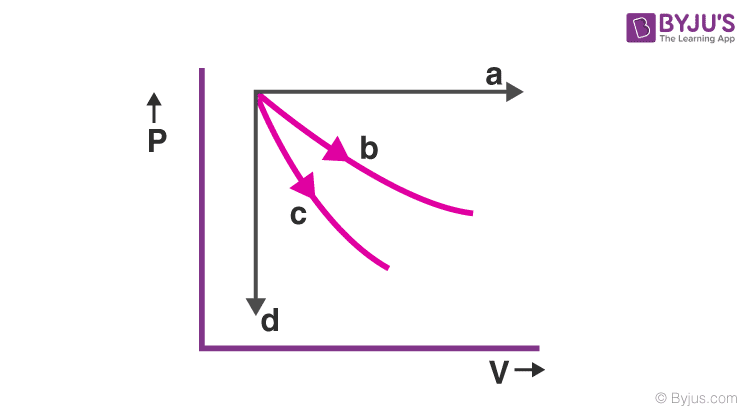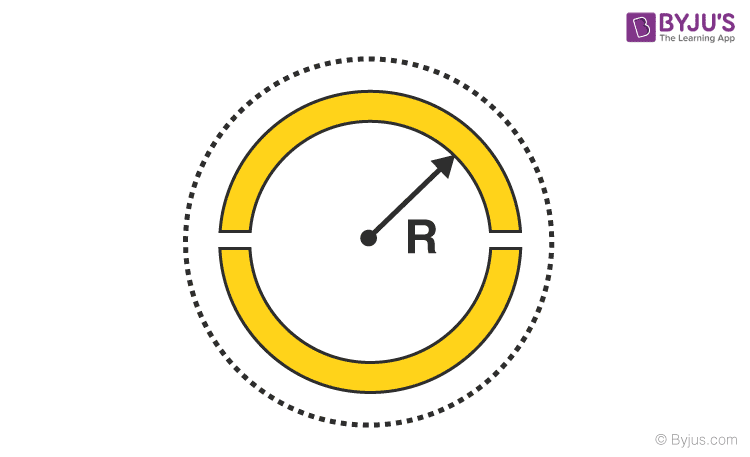# JEE Main Thermal Expansion Previous Year Questions with Solutions

The property of a body by which there is a change in the dimension like length, area or volume due to a change in temperature is called thermal expansion. The thermal expansion of a body can be studied as a function of temperature.

One of the common examples of thermal expansion is seen while opening a tight metal jar lid. People often loosen a tight metal jar lid by holding it under hot water. By doing so, the metal of the lid and the glass of the jar, both expand because the hot water adds energy to their atoms. But the metal expands more as compared to glass and gets loosened up.

Depending on the type of expansion thermal expansion is of 3 types– Linear expansion, Area expansion and Volume expansion. The expansion in length is called Linear Expansion. If a square tile is heated there is an expansion in length and breadth, so it is called Area Expansion. If a cube is taken and heated up all its sides expand and now the body experiences an increase in the overall volume due to this and it is called Volume Expansion.

## JEE Main Previous Year Solved Questions on Thermal Expansion

Q1: The given diagram shows four processes i.e., isochoric, isobaric, isothermal and adiabatic. The correct assignment of the processes, in the same order, is given by(a) d a c b

(b) d a b c

(c) a d b c

Solution

In an isochoric process, volume remains constant while in the isobaric process, pressure remains the same. The slope of an isothermal process is given as P/V while for an adiabatic process, the slope of the P – V curve is -γP/V, where γ >1. The adiabatic curve is steeper than the isothermal curve.

Answer: (b) d a b c

Q2: The ratio of work done by an ideal monoatomic gas to the heat supplied to it in an isobaric process is

(a) 2/5

(b) 3/2

(c) 3/5

(d) 2/3

Solution

For an ideal gas in an isobaric process,

Heat supplied, Q = nCpΔT

Work done, W = PΔV = nRΔT

Required Ratio = W/Q

Required Ratio = (nRΔT)/(nCpΔT) = R/[(5/2)R] = 2/5

(For monoatomic gas, Cp = (5/2)R

Q3: The work of 146 kJ is performed in order to compress one-kilo mole of gas adiabatically and in this process the temperature of the gas increases by 7ºC. The gas is (R = 8.3 J mol–1 K–1)

(a) monoatomic

(b) diatomic

(c) triatomic

(d) a mixture of monoatomic and diatomic

Solution

According to first law of thermodynamics

ΔQ = ΔU + ΔW

For an adiabatic process,ΔQ = 0

0 = ΔU + ΔW or ΔU = –Δ W

or nCVΔT = –ΔW

CV = –ΔW/nΔT

CV = -(-146) x 103/(1×103)x7 = 20.8 mol-1K-1

For diatomic gas, CV =(5/2)R = (5/2) x 8.3 = 20.8 Jmol-1K-1

Hence the gas is diatomic

Q4: Two moles of an ideal monatomic gas occupies a volume V at 27°C. The gas expands adiabatically to a volume 2V. Calculate (i) the final temperature of the gas and (ii) change in its internal energy.

(a) (i) 198 K (ii) 2.7 kJ

(b) (i) 195 K (ii) 2.7 kJ

(c) (i) 189 K (ii) 2.7 kJ

(d) (i) 195 K (ii) 2.7 kJ

Solution

For an adiabatic process, PVγ = constant

(nRT/V)Vγ = constant or TVγ – 1 = constant

T1V1γ – 1 = T2V2γ – 1

T2 = T1 [V1 / V2]γ – 1

Here, T1 = 27°C = 300 K, V1 = V, V2 = 2V, γ = 5/3

T2 = 300(V/2V)(5/3 – 1) = 300 (½)2/3 = 189K

Change in internal energy, ΔU = nCVΔT

ΔU = n[(f/2)R](T2 – T1) = 2 x (3/2) x (25/3)(189 – 300)

ΔU = – 2.7 kJ

Answer: (c) (i) 189 K (ii) 2.7 kJ

Q5: A wooden wheel of radius R is made of two semicircular parts (see figure). The two parts are held together by a ring made of a metal strip of cross-sectional area S and Length L. L is slightly less than 2πR. To fit the ring on the wheel, it is heated so that its temperature rises by ΔT and it just steps over the wheel. As it cools down to the surrounding temperature, it presses the semicircular parts together. If the coefficient of linear expansion of the metal is α, and it’s Young’s modulus is Y, the force that one part of the wheel applies on the other part is(a) 2SYαΔT

(b) 2πSYαΔT

(c) SYαΔT

(d) πSYαΔT

Solution

Increase in length, ΔL = LαΔT

ΔL/L= αΔT

The thermal stress developed is

T/S = Y(ΔL/L)

or T = SYαΔT

From FBD of one part of the wheel, F = 2T

Where F is the force that one part of the wheel applied to the other part.

F = 2SY αΔT

Q6: The pressure that has to be applied to the ends of a steel wire of length 10 cm to keep its length constant when its temperature is raised by 1000C is

(For steel Young’s modulus is 2 x 1011 Nm-2 and coefficient of thermal expansion is 1.1 x 10-5 K-1)

(a) 2.2 x 107 Pa

(b) 2.2 x 106 Pa

(c) 2.2 x 108 Pa

(d) 2.2 x 109 Pa

Solution

Given, ΔT = 100°C, Y = 2 × 1011 N m–2

α= 1.1 × 10–5 K–1

Thermal strain in the wire = αΔT [As l = l0 (1 +αΔT)]

Thermal stress in a rod is the pressure due to the thermal strain.

Required pressure = YαΔT = 2 × 1011 × 1.1 × 10–5 × 100 = 2.2 × 108 Pa

Answer: (c) 2.2 x 108 Pa

Q7: Two wires are made of the same material and have the same volume. However, wire 1 has cross-sectional area A and wire 2 has cross-sectional area 3A. If the length of wire 1 increases by Dx on applying force F, how much force is needed to stretch wire 2 by the same amount?

(a) 6F

(b) 9F

(c) F

(d) 4F

Solution

For the same material, Young’s modulus is the same and it is given that the volume is the same and the area of the cross-section for the wire l1 is A and that of l2 is 3A.

V = V1 = V2

V = A × l1 = 3A × l2

l2 = l1/3

Y = (F/A)/(Δl/l) ⇒ F1 = YA(Δl1/l1)

F1 = Y3A(Δl2/l2)

Given Δl1 = Δl2 = Δx (for the same extension)

F2 = Y3A(Δx /l1/3) = 9(YAΔx/l1) = 9F1 or 9F

Q8: A uniform cylindrical rod of length L and radius r, is made from a material whose Young’s modulus of elasticity equals Y. When this rod is heated by temperature T and simultaneously subjected to a net longitudinal compressional force F, its length remains unchanged. The coefficient of volume expansion, of the material of the rod, is (nearly) equal to

(a) 9F/(r 2YT)

(b) F/(3r 2YT)

(c) 6F/(r 2YT)

(d) 3F/(r 2YT)

Solution

Given, Length = L

Longitudinal force = F

Young’s modulus = Y

Temperature = T

Since length remains same \

(Stress)Compressive = (Stress)Thermal

F/A = YαT ⇒ F/πr2 = YαT (where = coeficient of linear expansion) 2 F r YT

α = F/πr2YT

Coefficient of volume expansion,γ = 3α

γ = 3F/πr2YT

Q9: The pressure that has to be applied to the ends of a steel wire of length 10 cm to keep its length constant when its temperature is raised by 100°C is (For steel Young’s modulus is 2 × 1011 N m–2 and coefficient of thermal expansion is 1.1 × 10–5 K–1)

(a) 2.2 × 106 Pa

(b) 2.2 × 108 Pa

(c) 2.2 × 109 Pa

(d) 2.2 × 107 Pa

Solution

Given, ΔT = 100°C, Y = 2 × 1011 N m–2

α = 1.1 × 10–5 K–1

Thermal strain in the wire =αΔT [As l = l0 (1 +αΔT)]

Thermal stress in the rod is the pressure due to the thermal strain.

Required pressure = YαΔT = 2 × 1011 × 1.1 × 10–5 × 100 = 2.2 × 108 Pa.

Answer: (b) 2.2 × 108 Pa

Q10: During an adiabatic process, the pressure of a gas is found to be proportional to the cube of its absolute temperature. The ratio CP /CV for the gas is

(a) 4/3

(b) 2

(c) 5/3

(d) 3/2

Solution

In an adiabatic process, Tγ = (constant)Pγ-1

Tγ/γ-1 = (constant)P

Given T3 = (constant) P

γ/γ-1 = 3 ⇒3γ – 3 =γ ⇒ γ =3/2

Q11: An external pressure P is applied on a cube at 0°C so that it is equally compressed from all sides. K is the bulk modulus of the material of the cube and is its coefficient of linear expansion. Suppose we want to bring the cube to its original size by heating. The temperature should be raised by

(a) 3/PαK

(b) P/3αK

(c) 3α/PK

(d) 3PKα

Solution

The bulk modulus of the gas is given by K = – P/(ΔV/V0)

ΔV/V0 = P/K……(1)

Also, V = V0(1 + γΔT) or ΔV/V0 = γΔT ……(2)

Comparing eq. (1) and (2), we get P/K = γΔT

ΔT = P/ 3αK (since γ= 3α)

Q12: Steel wire of length ‘L’ at 40°C is suspended from the ceiling and then a mass “m” is hung from its free end. The wire is cooled down from 40°C to 30°C to regain its original length “L”.The coefficient of linear thermal expansion of the steel is 10-5/°C, Young’s modulus of steel is 1011 N/m2 and radius of the wire is 1 mm. Assume that L>> diameter of the wire. Then the value of “m” in kg is nearly

Solution

Y = mgL/Ae here e= αL Δt

Y = mgL/AαL Δt

Y = mg/AαΔt

1011 = [M x10]/[(22/7)x1x10-6x10-5 x10]

m = 3.14 ⋍3

Q13: A diatomic gas with rigid molecules does 10J of work when expanded at constant pressure. What would be the heat energy absorbed by the gas, in this process?

(a) 25 J

(b) 35 J

(c) 30 J

(d) 40 J

Solution

F = Cv/Cp = 1/γ = 1/(7/5) = 5/7

W/Q = 1 – (5/7) = 2/7

Q = (7/2)W = (7 x 10)/2 = 35J